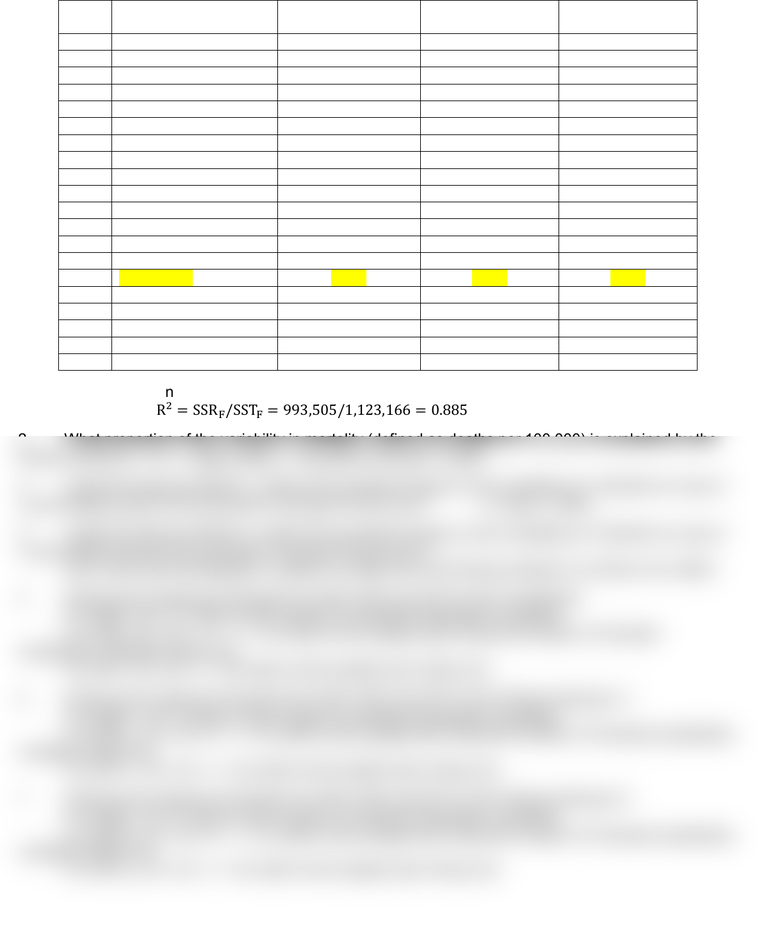Study Guides (380,000)
CA (150,000)
U of A (4,000)
MGTSC (10)
Quiz

# MGTSC312 Study Guide - Quiz Guide: Coefficient Of Determination, F 17 Kallinge, Dependent And Independent VariablesExam

Department
Management Science
Course Code
MGTSC312
Professor
Alice Nakamura
Study Guide
Quiz

This preview shows half of the first page. to view the full 2 pages of the document.1
Dependent variable: Mortality defined as deaths per 100,000.
Full Model
(F)
Reduced Model 1
(R1)
Reduced Model 2
(R2)
1
Intercept
847.6
929.1
725.6
2
SulphMin
0.5
2.2
3
SulphMean
-0. 5
-0.2
4
SulphMax
0.2
0.6
5
PartMin
-1.2
-2.1
6
PartMean
1.3
0.06
7
PartMax
-0.07
0.11
8
Population
-0.00002
-1.66E-05
9
PopDensity
0.05
0.06
10
%White
-5.4
-3.4
11
%NonPoor
-3.5
-5.4
12
%65+
83.8
84.9
Multiple R
0.941
0.911
0.641
R Square
0.885
0.830
0.411
0.839
0.805
0.304
13
Standard Error
68.049
74.931
141.551
14
Observations
40
40
40
15
SSR
993,505
932,269
461,951
16
SSE
129,660
190,897
661,214
17
SST
1,123,166
1,123,166
1,123,166
1. What proportion of the variability in mortality (defined as deaths per 100,000) that is explained by
the Full Model?  
2. What proportion of the variability in mortality (defined as deaths per 100,000) is explained by the
Reduced Model 1?   
3. Using the Reduced Model 1, what is the predicted impact on the mortality per 100,000 of a rise of
2 percentage points in the proportion of people 65 and over?  
4. Using the Reduced Model 2, what is the predicted impact on the mortality per 100,000 of a rise of
2 percentage points in the proportion of people 65 and over?
Zero, since that demographic variable is omitted from the Reduced Model 2 so there is no effect.
5. What are the degrees of freedom for SSR, SSE and SST for the Full Model?
For SSRF, df = 11, which is the number of included explanatory variables.
For SSEF, df = 40 11 1 = 28, which is the sample size minus the number of included
explanatory variables minus one.
For SSTF, df = 40 1 = 39, which is the sample size minus one.
6. What are the degrees of freedom for SSR, SSE and SST for the Reduced Model 1?
For SSRR1, df = 5, which is the number of included explanatory variables.
For SSE R1, df = 40 5 1 = 34, which is the sample size minus the number of included explanatory
variables minus one.
For SST R1, df = 40 1 = 39, which is the sample size minus one.
7. What are the degrees of freedom for SSR, SSE and SST for the Reduced Model 2?
For SSRR2, df = 6, which is the number of included explanatory variables.
For SSE R2, df = 40 6 1 = 33, which is the sample size minus the number of included explanatory
variables minus one.
For SST R2, df = 40 1 = 39, which is the sample size minus one.